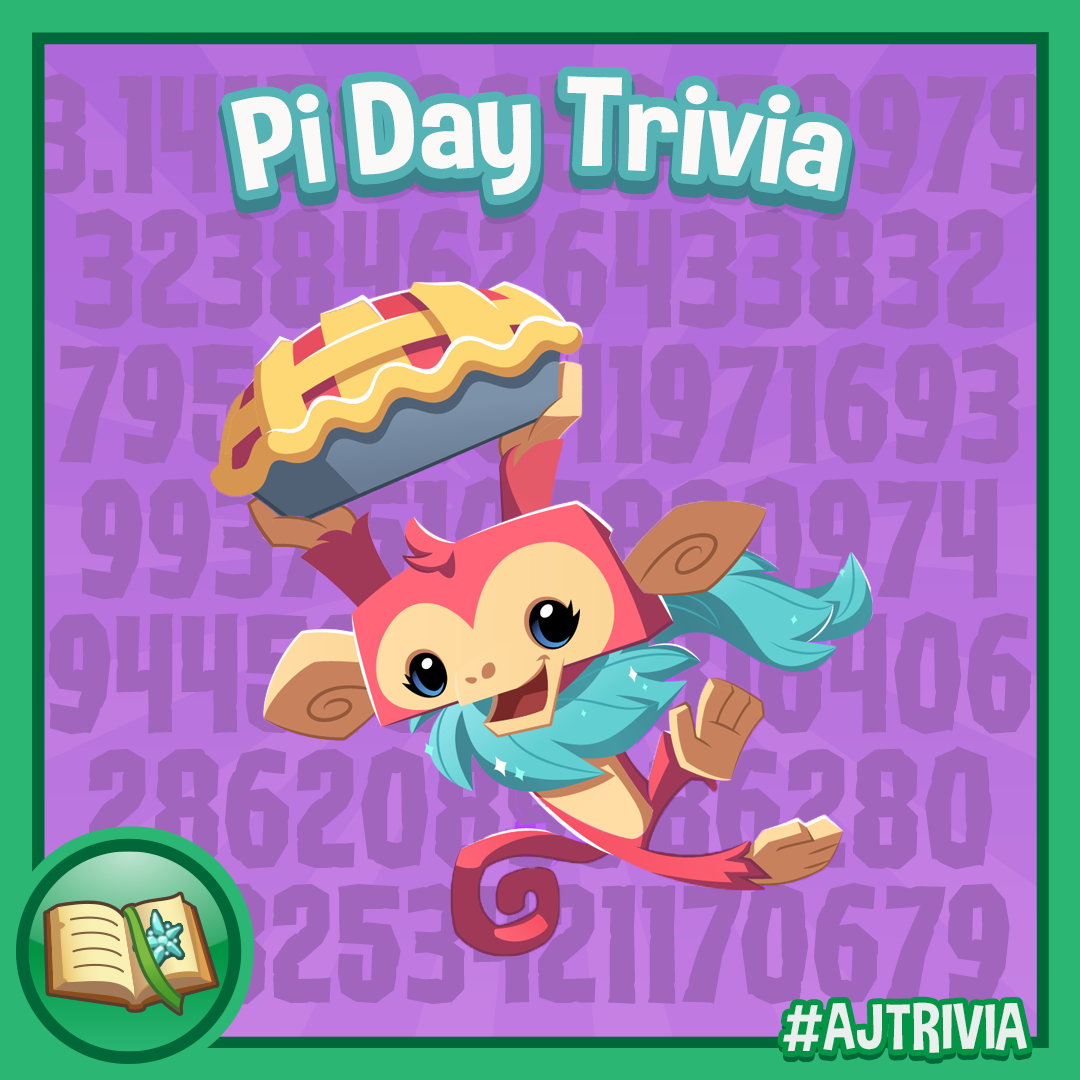##Pi Day Trivia!Are you ready for some Pi…we mean Pi Trivia!

What is the final digit of pi? A) 3 B) 1 C) 4 D) It can’t be calculated!

In mathematics, pi is known as: A) A rational number B) An irrational number C) A visible number D) An invisible number

Instead of pi, some mathematicians like using tau. A) Tau = the square root of 2 B) Tau = e C) Tau = 2π D) Tau doesn’t exist!